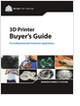Design HomeSpringsOverviewDesign ConsiderationsSpring BucklingExtension SpringsNatural FrequencyCalculatorsOverviewSpring ForceDeformed LengthSpring Rate kForce & StressRange of Rates kSpring DesignerSpring Designer EquationsCyclic Loads: ResonanceCyclic Loads: FatigueFatigue EquationsResourcesBibliography
3D Scanners

A white paper to assist in the evaluation of 3D scanning hardware solutions.

Essentials of Manufacturing

Information, coverage of important developments and expert commentary in manufacturing.

Selecting the Right 3D Printer

Discover how to choose the right 3D printer for your needs and the key performance attributes to consider.

CNC Machining Design Guide

more free publicationsFirst Natural Frequency FormulaWhen springs are used in a moving mechanism, their dynamic behaviors have to be analyzed. For example, valve springs in an engine have their own natural frequencies. The designers must ensure that springs are operated well under their first natural frequency at maximum engine speed, or they risk damage to the pistons because the springs may not return the valves in time. The first natural frequency of a helical spring is found to be,where d is the wire diameter, D is the nominal coil diameter, nt is the total number of coils, G and r are the shear modulus and density of the spring material, respectively.
Derivation By AnalogyAn easy way to derive the above equation is to draw an analogy between a rod and a spring. The analogy works because both objects are continuously distributed elements, in that their stiffness and mass are spread uniformly throughout their interiors.Both the spring and the rod obey Hooke's Law when used in static applications,where DL is the change in length of the spring or rod. The stiffness k for the rod is given by,where E is the Youngs modulus of the material, and A and L are defined in the picture above. The change in length of the rod for dynamic applications is given by,where the wavenumber n is given by,and f is the driving frequency (in Hz). To check that we have the right equation, we note 2 things: The dynamic equation for DL satisfies the governing partial differential equation for the rod, and DL becomes Hooke's Law in the static limit (f goes to zero),To find the natural frequencies for the rod, we look at where the change in length of the rod blows up. This occurs when nL in the denominator equals one of the following: {p, 2p, 3p, ...}. The first natural frequency occurs when,We solve for fres and substitute in krod and the volume of the rod (A*L),We recognize that the density times the volume equals the mass of the rod. We can therefore simplify the resonant frequency formula to,By analogy, the spring's first natural frequency will have the same equation,where k is now the spring stiffness, and M is the spring mass (which can be found by weighing the spring). Note that this equation is similar to that for a lumped spring-mass oscillator. However, the frequency here is a factor of p higher due to the distributed mass in the spring (and rod).
Expressed in Terms of Spring GeometryWe can express the natural frequency for the spring in terms of its geometry and shear modulus (instead of its overall stiffness k and its mass). To do so, we find the volume of the spring,and note the stiffness of the spring in terms of its geometry and shear modulus G and number of active coils na,Substituting these two equations into the formula for fres gives,If the spring has several coils, we can assume that the number of active coils equals the number of total coils. We can also allow the following numerical approximation,These two approximations give us our final equation for the spring resonant frequency,To use this formula we need to know the material's G and all of the spring geometry. It's much easier to use the formula from the last section which only needs the spring stiffness and mass, especially when working with springs where the material is uncertain.
Glossary3D Scanners

A white paper to assist in the evaluation of 3D scanning hardware solutions.Essentials of Manufacturing

Information, coverage of important developments and expert commentary in manufacturing.Selecting the Right 3D Printer

Discover how to choose the right 3D printer for your needs and the key performance attributes to consider.CNC Machining Design Guide